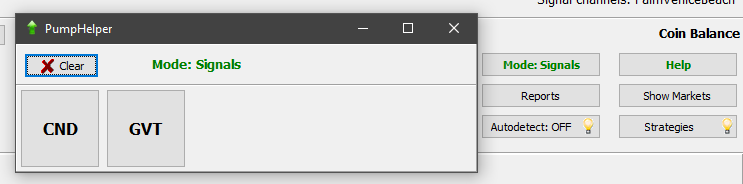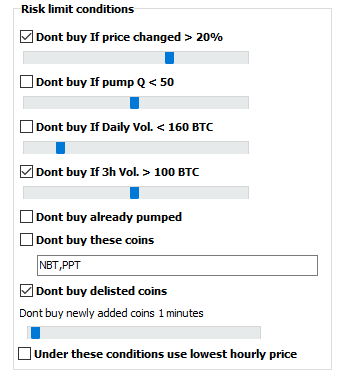### Statistics

Blog

Release 3.43 brings new feature - the "Pump Helper" tool. Use it to play pumps where the coin is given in a picture (so a bot can't directly read it, only human can).

How it works: first you should configure the pump detector strategy (settings example at the bottom of this page). Configure it such way that it gives about 3-5 signals per minute. You MUST turn off autobuy in the strategy also you may turn off auto opening charts.

Then activate the strategy, turn ON "autodetect", go to the Menu and select "Pump Tool". This brings the window with market buttons which the detector has found:Of course there will be false detections, thats why you turned OFF the autobuy option.

Now all you have to do is wait the pump channel and as soon as you see the coin, push appropriate button in the Pump Helper. In the "signals" mode this will immediately buy the coin. In the "manual" mode the button only opens the chart.

Note: the button is NOT related to the pump detetor strategy settings; its just a button to help you faster buy the coin. The settings it uses for order's handling are the settings from the main window !

When preparing to a pump pay attention to the following settings:

1. In the main window its strongly recommended  to use "buy for 30-secs old price", this will use limit order related to initial coin's price. Otherwise using market price is way too risky!2. Settings -> Main: For well-know pumps you may turn off all checkboxes in the picture below:If you don't know whether to trust a pump group, use these settings to prevent the bot from entering prepumped coins: "Dont buy If price changed more then 10%..15%", "If 3h volume more then like 50 BTC" and so on.

IMPORTANT: If the bot has not bought the coin (due to risk limit settings or the prise raised up as hell and you missed it)  - cancel your order and relax, or at least move the order down, but NEVER move it up!

The pump detector strategy example: (If you want less sensitivity, adjust the following:)

PumpPriceRaise=0.3000 (use higher value like 0.5 .. 1%)
PumpBuysPerSec=3
PumpVolPerSec=0.2000 (use higher value like 1-2 BTC for strong pumps)
PumpBuyersPerSecMin=1
PumpBuyersPerSecMax=40 (use lower value like 4-10)
PumpVolEMA=4.000 (2 instead of 4)

##Begin_Strategy
Active=-1
StrategyName=Binance Pump Working
Comment=
SignalType=PumpsDetection
ChannelName=
ChannelKey=
SilentNoCharts=YES
ReportToTelegram=NO
SoundAlert=NO
SoundKind=
KeepAlert=60
CoinsWhiteList=
CoinsBlackList=NBT
MinVolume=0
MaxVolume=3000
MinHourlyVolume=0
MaxHourlyVolume=150
PenaltyTime=300
Delta_3h_Min=0.0000
Delta_3h_Max=50.00
NextDetectPenalty=30
AutoBuy=NO
MaxActiveOrders=1
AutoCancelBuy=25
AutoCancelLowerBuy=60
BuyType=Buy
OrderSize=0.0000
buyPrice=4.000
Use30SecOldASK=YES
TlgUseBuyDipWords=YES
TlgBuyDipPrice=-10.5000
SellPrice=10.000
PriceDownTimer=0
PriceDownDelay=10
PriceDownPercent=0.2000
PriceDownRelative=NO
PriceDownAllowedDrop=0.1000
UseSignalStops=YES
UseStopLoss=NO
StopLossDelay=0
StopLoss=2.000
StopLossSpread=0.8000
AllowedDrop=0.0000
UseSecondStop=NO
TimeToSwitch2Stop=120
SecondStopLoss=2.000
UseStopLoss3=NO
TimeToSwitchStop3=60
StopLoss3=3.000
AllowedDrop3=1.0000
UseTrailing=NO
TrailingPersent=-1.0000
TrailingSpread=0.8000
UseTakeProfit=NO
TakeProfit=1.500
DropsMaxTime=600
DropsPriceMA=10
DropsLastPriceMA=1
DropsPriceDelta=2.000
DropsPriceIsLow=NO
WallsMaxTime=0
WallsPriceDelta=0.0000
WallBuyVolDeep=0.0000
WallBuyVolume=0
WallBuyVolToDailyVol=0.0000
WallSellVolToBuy=0.0000
WallSellVolDeep=0.0000
PumpPriceRaise=0.3000
PumpBuysPerSec=3
PumpVolPerSec=0.2000
PumpBuyersPerSecMin=1
PumpBuyersPerSecMax=40
PumpVolEMA=4.000
PumpBuyersInterval=4.000
PumpMoveTimer=3
PumpMovePersent=0.5000
MShotPriceMin=2.000
MShotPrice=7.000
MShotAddHourlyDelta=0.1000
MShotSellAtLastPrice=YES
MShotSellPriceAdjust=0.0000
MShotReplaceDelay=0.0000
MShotRaiseWait=30.00
MShotSortBy=Last2hDelta
VolShortInterval=5
VolShortPriseRaise=0.5000
VolLongInterval=1800
VolBvShortToLong=3.000
VolBvLongToHourlyMin=0.2000
VolBvLongToHourlyMax=2.000
VolBvLongToDailyMin=0.2000
VolBvLongToDailyMax=2.000
VolBvToSvShort=5.000
VolBvShort=0.5000
VolBuyersShort=3
VolSvLong=0.4000
VolTakeLongMaxP=NO
VolAtMinP=1.500
VolAtMaxP=0.5000
VolDeltaAtMaxP=0.5000
VolDeltaAtMinP=0.2000
volBidsDeep=3.000
volBids=0.5000
volAsksDeep=4.000
volBidsToAsks=1.500
VLiteT0=300
VLiteT1=180
VLiteT2=180
VLiteT3=180
VLiteP1=1.0000
VLiteP2=1.0000
VLiteP3=1.0000
VLiteMaxP=5.000
VLitePDelta1=0.0000
VLitePDelta2=0.0000
VLiteDelta0=2.000
VLiteMaxSpike=7.000
VLiteV1=1.0000
VLiteV2=1.0000
VLiteV3=1.0000
VLiteWeightedAvg=YES
VLiteReducedVolumes=NO
WavesT0=300
WavesT1=180
WavesT2=180
WavesT3=180
WavesP1=1.0000
WavesP2=-11.0000
WavesP3=1.0000
WavesDelta0=2.000
WavesMaxSpike=7.000
WavesV1=1.0000
WavesV2=1.0000
WavesV3=1.0000
WavesWeightedAvg=YES
WavesReducedVolumes=NO
DeltaInterval=600
DeltaShortInterval=5
DeltaPrice=2.000
DeltaVol=5.000
DeltaVolRaise=2.000
DeltaVolSec=0.1000
DeltaBuyers=15
DeltaLastPrice=0.3000
ComboStart=
ComboEnd=
ComboDelayMin=0
ComboDelayMax=600
##End_Strategy#